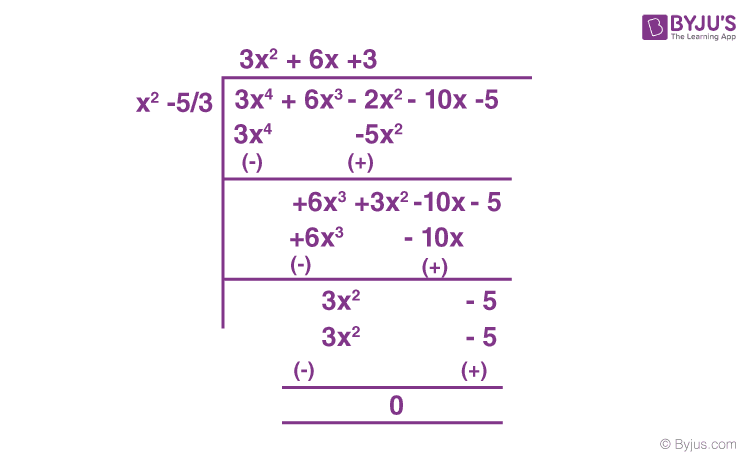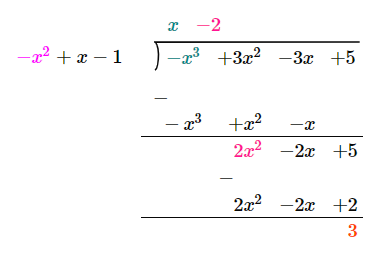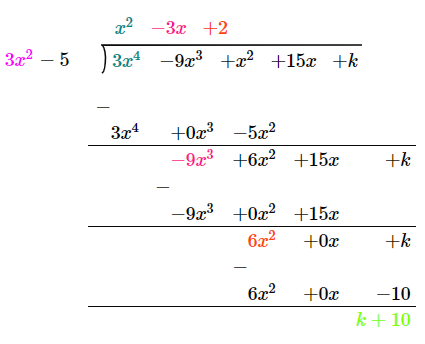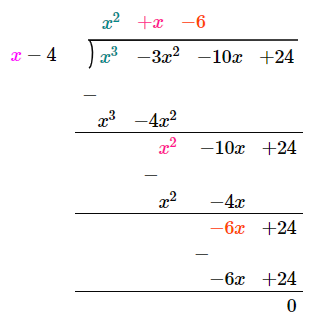# Important Questions Class 10 Maths Chapter 2 Polynomials

Important questions for Class 10 Maths Chapter 2 Polynomials are provided here as per the NCERT book. These questions are provided with answers that will help the students prepare and score well in the CBSE Class 10 Maths exam. The questions here cover the latest syllabus as prescribed by the board. Also, they are based on the latest exam pattern. Students can practice the important questions for all the chapters of 10th standard Maths subject and prepare well for the board exam. Along with the important questions, students will also find detailed solutions. In case they get stuck while solving the questions, then they can refer to the solutions.

We have also provided some additional important questions from the examination point of view for Chapter 2 Polynomials of Class 10 Maths so that students can revise the chapter by solving these problems instantly. This chapter deals with expressions carrying unknown variables with different degrees. The solutions of these expressions are termed zeros of the polynomial. The polynomial with degree 1 is the linear polynomial, with degree 2 is a quadratic polynomial, with degree 3 is a cubic polynomial.

Students can also get access to Class 10 Maths Chapter 2 Polynomials MCQs here.

Also Check:

## Class 10 Maths Chapter 2 Important Questions With Solutions

A few important Class 10 polynomials questions are provided below with solutions. These questions include both short and long answer questions to let the students get acquainted with the in-depth concepts.

Q.1: Find the value of “p” from the polynomial x2 + 3x + p, if one of the zeroes of the polynomial is 2.

Solution:

As 2 is the zero of the polynomial.

We know that if α is a zero of the polynomial p(x), then p(α) = 0

Substituting x = 2 in x2 + 3x + p,

⇒ 22 + 3(2) + p = 0

⇒ 4 + 6 + p = 0

⇒ 10 + p = 0

⇒ p = -10

Q.2: Does the polynomial a4 + 4a2 + 5 have real zeroes?

Solution:

In the aforementioned polynomial, let a2 = x.

Now, the polynomial becomes,

x2 + 4x + 5

Comparing with ax2 + bx + c,

Here, b2 – 4ac = 4– 4(1)(5) = 16 – 20 = -4

So, D = b2 – 4ac < 0

As the discriminant (D) is negative, the given polynomial does not have real roots or zeroes.

Q.3: Compute the zeroes of the polynomial 4x2 – 4x – 8. Also, establish a relationship between the zeroes and coefficients.

Solution:

Let the given polynomial be p(x) = 4x2 – 4x – 8

To find the zeroes, take p(x) = 0

Now, factorise the equation 4x2 – 4x – 8 = 0

4x2 – 4x – 8 = 0

4(x2 – x – 2) = 0

x2 – x – 2 = 0

x2 – 2x + x – 2 = 0

x(x – 2) + 1(x – 2) = 0

(x – 2)(x + 1) = 0

x = 2, x = -1

So, the roots of 4x2 – 4x – 8 are -1 and 2.

Relation between the sum of zeroes and coefficients:

-1 + 2 = 1 = -(-4)/4 i.e. (- coefficient of x/ coefficient of x2)

Relation between the product of zeroes and coefficients:

(-1) × 2 = -2 =  -8/4 i.e (constant/coefficient of x2)

Q.4: Find the quadratic polynomial if its zeroes are 0, √5.

Solution:

A quadratic polynomial can be written using the sum and product of its zeroes as:

x2 – (α + β)x + αβ

Where α and β are the roots of the polynomial.

Here, α = 0 and β = √5

So, the polynomial will be:

x2 – (0 + √5)x + 0(√5)

= x2 – √5x

Q.5: Find the value of “x” in the polynomial 2a2 + 2xa + 5a + 10 if (a + x) is one of its factors.

Solution:

Let f(a) = 2a2 + 2xa + 5a + 10

Since, (a + x) is a factor of 2a2 + 2xa + 5a + 10, f(-x) = 0

So, f(-x) = 2x2 – 2x2 – 5x + 10 = 0

-5x + 10 = 0

5x = 10

x = 10/5

Therefore, x = 2

Q.6: How many zeros does the polynomial (x – 3)2 – 4 have? Also, find its zeroes.

Solution:

Given polynomial is (x – 3)2 – 4

Now, expand this expression.

=> x2 + 9 – 6x – 4

= x2 – 6x + 5

As the polynomial has a degree of 2, the number of zeroes will be 2.

Now, solve x2 – 6x + 5 = 0 to get the roots.

So, x2 – x – 5x + 5 = 0

=> x(x – 1) -5(x – 1) = 0

=> (x – 1)(x – 5) = 0

x = 1, x = 5

So, the roots are 1 and 5.

Q.7: α and β are zeroes of the quadratic polynomial x2 – 6x + y. Find the value of ‘y’ if 3α + 2β = 20.

Solution:

Let, f(x) = x² – 6x + y

From the given,

3α + 2β = 20———————(i)

From f(x),

α + β = 6———————(ii)

And,

αβ = y———————(iii)

Multiply equation (ii) by 2. Then, subtract the whole equation from equation (i),

=> α = 20 – 12 = 8

Now, substitute this value in equation (ii),

=> β = 6 – 8 = -2

Substitute the values of α and β in equation (iii) to get the value of y, such as;

y = αβ = (8)(-2) = -16

Q.8: If the zeroes of the polynomial x– 3x+ x + 1 are a – b, a, a + b, then find the value of a and b.

Solution:

Let the given polynomial be:

p(x) = x– 3x+ x + 1

Given,

The zeroes of the p(x) are a – b, a, and a + b.

Now, compare the given polynomial equation with general expression.

px+ qx2 + rx + s = x– 3x2 + x + 1

Here, p = 1, q = -3, r = 1 and s = 1

For sum of zeroes:

Sum of zeroes will be = a – b + a + a + b

-q/p = 3a

Substitute the values q and p.

-(-3)/1 = 3a

a = 1

So, the zeroes are 1 – b, 1, 1 + b.

For the product of zeroes:

Product of zeroes = 1(1 – b)(1 + b)

-s/p = 1 – 𝑏2

=> -1/1 = 1 – 𝑏2

Or, 𝑏2 = 1 + 1 =2

So, b = √2

Thus, 1 – √2, 1, 1 + √2 are the zeroes of equation 𝑥3 − 3𝑥2 + 𝑥 + 1.

Q.9: Find a quadratic polynomial each with the given numbers as the sum and product of its zeroes, respectively.

(i) 1/4, -1

(ii) 1, 1

(iii) 4, 1

Solution:

(i) From the formulas of sum and product of zeroes, we know,
Sum of zeroes = α + β
Product of zeroes = αβ

Given,

Sum of zeroes = 1/4
Product of zeroes = -1

Therefore, if α and β are zeroes of any quadratic polynomial, then the polynomial can be written as:-
x2 – (α + β)x + αβ

= x2 – (1/4)x + (-1)

= 4x2 – x – 4

Thus, 4x2 – x – 4 is the required quadratic polynomial.

(ii) Given,
Sum of zeroes = 1 = α + β
Product of zeroes = 1 = αβ

Therefore, if α and β are zeroes of any quadratic polynomial, then the polynomial can be written as:-

x2 – (α + β)x + αβ

= x2 – x + 1

Thus, x2 – x + 1 is the quadratic polynomial.

(iii) Given,
Sum of zeroes, α + β = 4
Product of zeroes, αβ = 1

Therefore, if α and β are zeroes of any quadratic polynomial, then the polynomial can be written as:-

x2 – (α + β)x + αβ

= x2 – 4x + 1

Thus, x2 – 4x +1 is the quadratic polynomial.

Q.10: Obtain all other zeroes of 3x+ 6x– 2x– 10x – 5, if two of its zeroes are √(5/3) and-√(5/3).

Solution: Since this is a polynomial of degree 4, hence there will be a total of 4 roots.

√(5/3) and-√(5/3) are zeroes of polynomial f(x).
∴ [x-√(5/3)] [x+√(5/3)] = x2-(5/3)Therefore, 3x+ 6x + 3 = 3x(x + 1) +3 (x + 1)
= (3x + 3)(x + 1)
= 3(x + 1)(x + 1)
= 3(x + 1)(x + 1)
Hence, x + 1 = 0 i.e. x = – 1 , – 1 is a zero of p(x).
So, its zeroes are given by: x = −1 and x = −1.
Therefore, all four zeroes of the given polynomial are:
√(5/3) and-√(5/3), −1 and −1.

Q.11: Find a quadratic polynomial whose zeroes are reciprocals of the zeroes of the polynomial f(x) = ax2 + bx + c, a ≠ 0, c ≠ 0.

Solution:

Let α and β be the zeroes of the polynomial f(x) = ax2 + bx + c.

So, α + β = -b/a

αβ = c/a

According to the given, 1/α and 1/β are the zeroes of the required quadratic polynomial.

Now, the sum of zeroes = (1/α) + (1/β)

= (α + β)/αβ

= (-b/a)/ (c/a)

= -b/c

Product of two zeroes = (1/α) (1/β)

= 1/αβ

= 1/(c/a)

= a/c

The required quadratic polynomial = k[x2 – (sum of zeroes)x + (product of zeroes)]

= k[x2 – (-b/c)x + (a/c)]

= k[x2 + (b/c) + (a/c)]

Q.12: Divide the polynomial f(x) = 3x2 – x3 – 3x + 5 by the polynomial g(x) = x – 1 – x2 and verify the division algorithm.

Solution:

Given,

f(x) = 3x2 – x3 – 3x + 5

g(x) = x – 1 – x2

Dividing f(x) = 3x2 – x3 – 3x + 5 by g(x) = x – 1 – x2Here,

Quotient = q(x) = x – 2

Remainder = r(x) = 3

By division algorithm of polynomials,

Dividend = (Quotient × Divisor) + Remainder

So,

[q(x) × g(x)] + r(x) = (x – 2)(x – 1 – x2) + 3

= x2 – x – x3 – 2x + 2 + 2x2 + 3

= 3x2 – x3 – 3x + 5

= f(x)

Hence, the division algorithm is verified.

Q.13: For what value of k, is the polynomial f(x) = 3x4 – 9x3 + x2 + 15x + k completely divisible by 3x2 – 5?

Solution:

Given,

f(x) = 3x4 – 9x3 + x2 + 15x + k

g(x) = 3x2 – 5

Dividing f(x) by g(x),Given that f(x) is completely divisible by 3x2 – 5.

So, the remainder = 0

k + 10 = 0

k = -10

Q.14: If 4 is a zero of the cubic polynomial x3 – 3x2 – 10x + 24, find its other two zeroes.

Solution:

Given cubic polynomial  is p(x) = x3 – 3x2 – 10x + 24

4 is a zero of p(x).

So, (x – 4) is the factor of p(x).

Let us divide the given polynomial by (x – 4).Here, the quotient = x2 + x – 6

= x2 + 3x – 2x – 6

= x(x + 3) – 2(x + 3)

= (x – 2)(x + 3)

Therefore, the other two zeroes of the given cubic polynomial are 2 and -3.

### Practice Questions for Class 10 Maths Chapter 2 Polynomials

1. Find the zeroes of the quadratic polynomial 7y2 – (11/3)y – (2/3) and verify the relationship between the zeroes and the coefficients.
2. Find all zeroes of the polynomial (2x4 – 9x3 + 5x2 + 3x – 1) if two of its zeroes are (2 + √3) and (2 – √3).
3. If the remainder on division of x3 + 2x2 + kx +3 by x – 3 is 21, find the quotient and the value of k. Hence, find the zeroes of the cubic polynomial x3 + 2x2 + kx – 18.
4. For which values of a and b, are the zeroes of q(x) = x3 + 2x2 + a also the zeroes of the polynomial p(x) = x5 – x4 – 4x3 + 3x2 + 3x + b? Which zeroes of p(x) are not the zeroes of q(x)?
5. Verify that 3, –1, -1/3 are the zeroes of the cubic polynomial p(x) = 3x3 – 5x2 – 11x – 3, and then verify the relationship between the zeroes and the coefficients.
6. Find a quadratic polynomial, the sum and product of whose zeroes are √2 and -3/2, respectively. Also find its zeroes.

1. Sakkhya Sinha

2. kavita

my doubt is of relationship between zeros ans coffieients

1. lavanya
2. relationship between zeroes and coefficients means that you have to show that alpha + beta = -b/a and alpha x beta = c/a.

3. Kanishq Garg

good questions

4. Tanishka

It was really useful

5. Manasvishnoi

Please tell me some tough questions???
These were too easy.

6. Riddhi

Ya! It is useful , good questions .

7. this all are so easy and nice thanks to byju’s

8. aneetta gitto

happy with these questions .. will be more happy if got hard questions and i need some difficult questions on relationship between zeroes and coefficient

9. shivani

its easy

10. mith jain

it is very helpfull questions my sons douts are all cleared . thank u

12. These questions were useful.

13. Aaryan singh

I need some hard question from this chapter

14. Thank you for the questions, these really helped me clear my doubts.

15. Very useful questions for revision

16. Maths slayer

Very easy but good question thanks byjus

17. Veryy amazing I just love it I hope byjus go through the sky 😃😃😃😃😃😃

18. Mohammed Abdul Raees

It’s very useful before examination for us like 10 std so thank you for posting questions like this every week